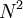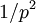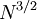Rouse model

The distance between two units separated by a sufficient number of bonds in a flexible polymer chain follows a Gaussian distribution. Therefore, the polymer chain can be described by a coarse-grained model of N successive Gaussian segments. A dynamical model is consistent with this description and also with Boltzmann's distribution of energies if it assumes that the forces between units jointed by the Gaussian segments are proportional to their distances (Hookean springs). The Rouse model  describes a polymer chain as a set of N coupled harmonic springs. The mathematical treatment of this model decomposes the global motion in a set of N-1 independent "normal modes". The time-correlation function of each one of these modes decays as a single exponential, characterized by a "Rouse relaxation time". The Rouse relaxation times are proportional to$N^2$ (N is proportional to the chain length or the polymer molecular weight). The first Rouse mode represents the slowest internal motion of the chain. The p-th Rouse relaxation time is proportional to$1/p^2$.
In dilute solution, the relaxation times obtained from the Rouse model are not correct due to the presence of hydrodynamic interactions between units, introduced by Zimm . With this correction, the Zimm-Rouse relaxation times are proportional to$N^{3/2}$. This result is only valid for unperturbed (or theta) solvents. For solvent of good quality, excluded volume interactions have also to be accounted. However, the scheme of normal modes is maintained . In non-dilute solutions and melts hydrodynamic interactions and excluded volume effects are screened out and the Rouse model is correct, though only in the scale of short times and distances.